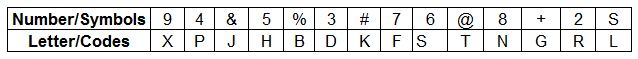### IBPS Clerk 15-Dec-2012 Question 187

Instructions

In each question below is given a group of numbers/symbols followed by five combinations of letter codes numbered a: , b:.c:. d: and e:. You have to find out which of the combinations correctly represents the group of numbers/symbols based on the following coding system and the conditions and mark the number of that combination as your answer.Conditions :
(i) If the first element is a symbol and the last element is a number, then the codes for both are to be interchanged.
(ii) If both the first and last elements are symbols, then the last element is to be coded as the code lor the first element.
(iii) If the group of elements contains only one symbol, then that symbols is to be coded as A.

Question 187

# @62+74

Solution

In the element, @62+74, the first letter is a symbol and the last is a number.

Thus, applying rule(i), the codes for '4' & '@' will be interchanged.

=> @62+74 - PSRGFT

• Banking Quant Shortcuts PDF
• Free Banking Study Material - (15000 Questions)
• 135+ Banking previous papers with solutions PDF